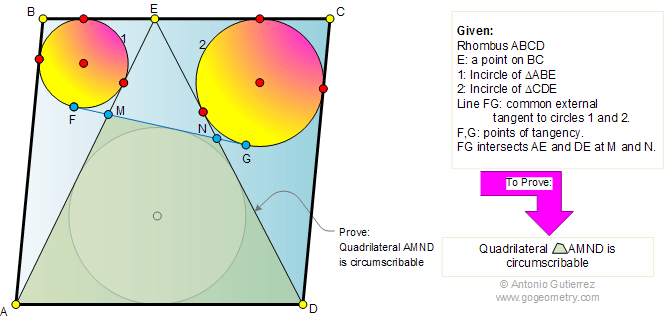Problem 351. Rhombus, Incircles, Common tangent, Circumscribable or Tangential quadrilateral. Level: High School, College, SAT Prep. The figure shows a rhombus ABCD with a point E on side BC. Circles 1 and 2 are the incircles of triangles ABE and CDE, respectively. FG is the common tangent to circles 1 and 2. FG intersects to AE and DE at M and N, respectively. Prove that the quadrilateral AMND is circumscribable or tangential (sides all lie tangent to a single circle inscribed within the quadrilateral).Recent Additions